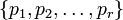# Exponent of a finite group equals product of exponents of its Sylow subgroups

Suppose$G$ is a finite group and$\{ p_1,p_2, \dots, p_r \}$ is the set of prime numbers dividing the order of$G$. For each$p_i$, let$P_i$ be a$p_i$-Sylow subgroup of$G$. Then, we have:$\mbox{Exponent of } G = \prod_{i=1}^r (\mbox{exponent of } P_i)$